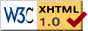CAOSP abstracts, Volume: 50, No.: 4, year: 2020

Abstract: The fundamental stages of development of the polytropic theory of stars with axial rotation are considered as a generalization of the Lane-Emden theory. The solution of the differential equilibrium equation for the polytropic star model with index n=1 and axial rotation with the angular velocity ω is presented in the form of infinite series of the Legendre polynomials and the spherical Bessel functions. Two variants of the approximate solution in the form of the finite number of terms are proposed. Integration constants were found in a self-consistent way using the integral form of the equilibrium equation and the iteration numerical method. Dependence of the geometrical and physical characteristics of the model on the dimensionless angular velocity Ω=ω(2π Gρc)-1/2 (where ρc is the density in the centre) is analyzed. A comparison with the results of other authors is performed. The obtained critical value of the angular velocity Ωmax, when an instability occurs is smaller than in other works (Chandrasekhar, 1933; James, 1964, and et al.). The inverse problem is also considered -- a determination of the polytropic model parameters for individual stars based on the solution of the equilibrium equation according to the values of their masses and radii, which are known from observations. In particular, the model parameters for the star α Eri, as well as a similar "class" of the star models of types O5÷G0, were determined. The solution of the equilibrium equation for the polytrope n=1+δ (where δ is a small value) is obtained using the method of perturbation theory.